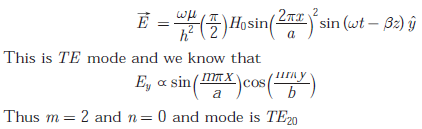# Communication Engineering - Online Test

Q1. Let the Laplace transform of a function F(t) which exists for t > 0 be F1(s) and the Laplace transform of its delayed version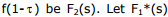be the complex conjugate of F1(s) with the Laplace variable set as s=σ + jw. If G(s) =, then the inverse Laplace transform of G(s) is
Explaination / Solution:Q2. During transmission over a certain binary communication channel, bit errors occur independently with probability p. The probability of AT MOST one bit in error in a block of n bits is given by
Explaination / Solution: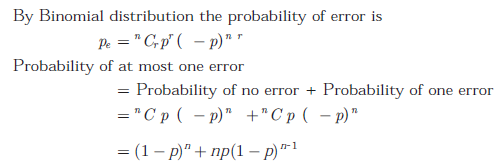Q3. A zero mean random signal is uniformly distributed between limits –a and +a and its mean square value is equal to its variance. Then the r.m.s value of the signal is
Explaination / Solution:Q4. In a GSM system, 8 channels can co-exist in 200 kHz bandwidth using TDMA. A GSM based cellular operator is allocated 5 MHz bandwidth. Assuming a frequency reuse factor of 1/5, i.e. a five-cell repeat pattern, the maximum number of simultaneous channels that can exist in one cell is
Explaination / Solution: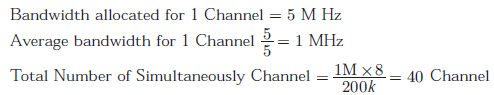Q5. The response h(t) of a linear time invariant system to an impulse δ(t) , under initially relaxed condition is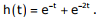The response of this system for a unit step input u(t) is
Explaination / Solution: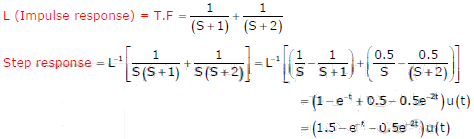Q6. In a Direct Sequence CDMA system the chip rate is 1.2288 × 106 chips per second. If the processing gain is desired to be AT LEAST 100, the data rate
Explaination / Solution:Q7. Given that f(y) = | y | / y, and q is any non-zero real number, the value of | f(q) - f(-q) | is
Explaination / Solution: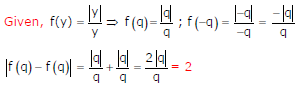Q8. An air-filled rectangular waveguide has inner dimensions of 3 cm × 2 cm. The wave impedance of the TEmode of propagation in the waveguide at a frequency of 30 GHz is (free space impedance η0 = 377Ω)
Explaination / Solution: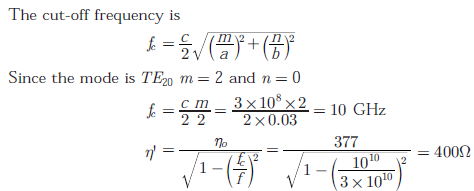Q9.
A periodic voltage waveform observed on an oscilloscope across a load is shown. A permanent magnet moving coil (PMMC) meter connected across the same load reads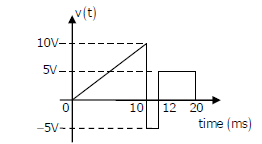Explaination / Solution:Q10. The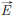field in a rectangular waveguide of inner dimension a × b is given byWhere H0 is a constant, and a and b are the dimensions along the x − axis and the y − axis respectively. The mode of propagation in the waveguide is
Explaination / Solution: